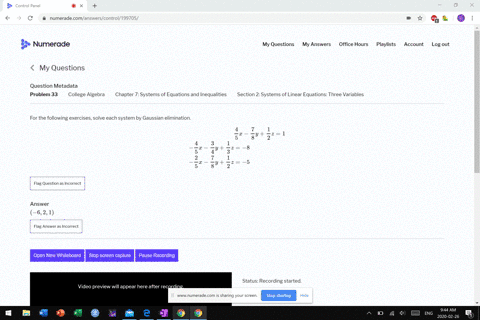Enroll in one of our FREE online STEM bootcamps. Join today and start acing your classes!View Bootcamps07:48
MB
Problem 32

# For the following exercises, solve each system by Gaussian elimination.$$\begin{array}{l}{\frac{1}{2} x-\frac{1}{4} y+\frac{3}{4} z=0} \\ {\frac{1}{4} x-\frac{1}{10} y+\frac{2}{5} z=-2} \\ {\frac{1}{8} x+\frac{1}{5} y-\frac{1}{8} z=2}\end{array}$$

## Discussion

You must be signed in to discuss.

## Video Transcript

No transcript available